Next: Continuous observations Up: Hidden Markov models Previous: Markov chains   Contents

## Hidden states

Hidden Markov model is basically a Markov chain whose internal state cannot be observed directly but only through some probabilistic function. That is, the internal state of the model only determines the probability distribution of the observed variables.

Let us denote the observations by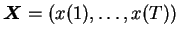. For each state, the distribution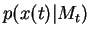is defined and independent of the time index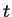. The exact form of this conditional distribution depends on the application. In the simplest case there is only a finite number of different observation symbols. In this case the distribution can be characterised by the point probabilities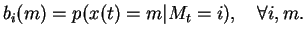(4.4)

Letting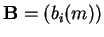and the parameters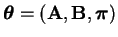, the joint probability of an observation sequence and a state sequence can be evaluated by simple extension to Equation (4.3)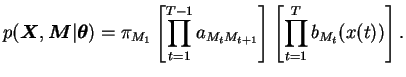(4.5)

The posterior probability of a state sequence can be derived from this as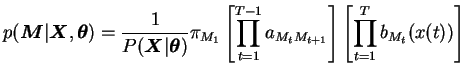(4.6)

where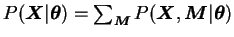.Next: Continuous observations Up: Hidden Markov models Previous: Markov chains   Contents
Antti Honkela 2001-05-30